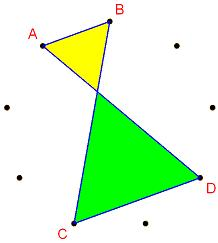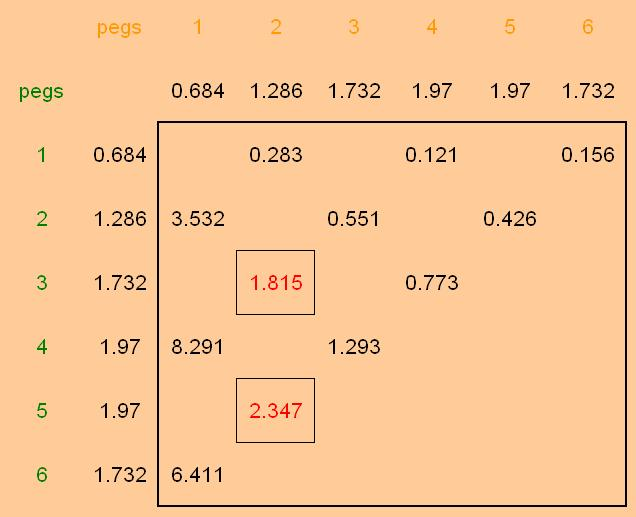#### You may also like### Coke Machine

The coke machine in college takes 50 pence pieces. It also takes a certain foreign coin of traditional design...### At a Glance

The area of a regular pentagon looks about twice as a big as the pentangle star drawn within it. Is it?### Cosines Rule

Three points A, B and C lie in this order on a line, and P is any point in the plane. Use the Cosine Rule to prove the following statement.

# Figure of Eight

##### Age 14 to 16Challenge Level

Well done Andrei from Tudor Vianu National College, Bucharest, and Michael from Portsmouth for sorting this one out.

There are nine gaps and the top and bottom lengths on the "Figure of Eight" must be parallel.

This means that the two sides, from top down to the bottom, must each cover the same number of pegs, because of the symmetry.

Those sides could be $1$, $2$, or $3$, but not $4$ because that only leaves one gap ($9-2\times4$) for top and bottom between them!

Working through those possibilities systematically:

$1$ for the sides leaves $7$ ($9 - 2$) for the top and bottom: $1$ with $6$, $2$ with $5$, or $3$ with $4$.

$2$ for the sides leaves $5$ ($9 - 4$) for the top and bottom : $1$ with $4$, or $2$ with $3$

$3$ for the sides leaves $3$ ($9 - 6$) for the top and bottom : so just $1$ with $2$

Between two adjacent pegs the angle at the centre is $40^\circ$

A chord between any two pegs and the centre of the pegboard makes an isosceles triangle.

Split that triangle along its line of symmetry to get a right-angled triangle and then use trigonometry to see that :

when the pegs are $n$ gaps apart, and the radius of the board is taken as $1$, the length of the chord is $2\sin{20n^\circ}$AB is parallel to CD which means that both the yellow and green triangles have the same set of angles.

So, because the yellow and green triangles are similar, the ratio of their areas is the square of the ratio for lengths.

And, from the paragraph above, we know that the side lengths can always be calculated.

$\frac{AREA CDE}{AREA ABE}$ near $2$ implies that ($\frac{CD}{AB})^2$ must be near 2Along the top are the number of gaps on the pegboard that $AB$ spans.

Down the side are the same for $CD$

Beneath or beside each of those are the lengths calculated using $2\sin{20n^\circ}$

The table shows the ($\frac{CD}{AB})^2$ values.

The combinations that do not make a Figure of Eight with $AB$ and $CD$ parallel are blanked out.

There are two values near $2$ but $1.815$ is the nearer so the solution is when yellow has two pegs and green has three pegs

Click here for a copy of the Excel file Figure of Eight Results